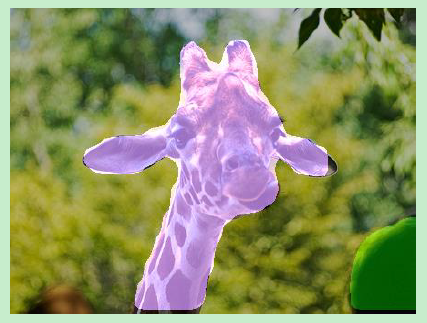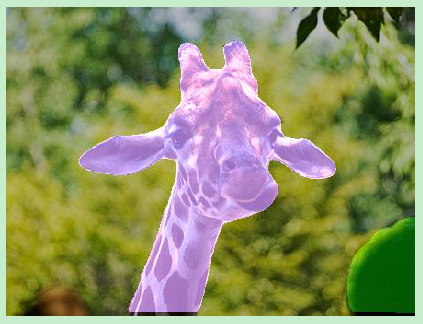arXiv-2019

# 1 Background and Motivation

• Proposal-based methods：先用目标检测方法检测目标框，再在框内区域进行 pix-level 的分类。代表性的方法有 Mask R-CNN （参考【Mask RCNN】《Mask R-CNN》） ， one-stage 普遍没有 two-stage 的目标检测方法的方法猛，two-stage 中 RoI pooling 操作又会存在如下两个缺点
• results in the loss of features and the distortion to the aspect ratios
• complex to adjust too many parameters
• segmentation based methods： segment first then do clustering，这类方法都没有 re-pooling 操作（RoI pooling），难点在 cluster 的过程中，很难去 determine the number of clusters or the positions of the cluster centers

# 2 Related Work

• Proposal-based methods：detection and segmentation
• Segmentation-based Methods（bottom-up methods）：first segmenting and then clustering（eg 让属于同一实例的像素在 embedding 空间中尽量靠在一起）

• propose a framework that unites the proposal-based and segmentation-based methods，通过 pixel-embedding 和 proposal embedding
• one-stage 实例分割方法，但是 higher quality（挑了些图） and higher speed than two-stage 的 Mask RCNN（但 AP 没别人高哟）

# 4 Method

pixel embeddings, proposal embeddings, and proposal margins to extract the instance masksd = 32 d=32

location x j x_j p r o p o s a l j proposal_j 的所有参数为 { c l a s s j , b o x j , c e n t e r j , q j , σ j } \{class_j, box_j,center_j, q_j,\sigma_j\}

σ j \sigma_j 是 proposal margin

pixel embedding 和 proposal embedding 的相似度来生成每个候选区域中的 mask，proposal margin δ \delta 相当于一个相似度的阈值，来决定最终的 mask

## 4.1 Embedding Definition• proposal embedding, which is a good representation of entire instance

• pixel embedding, which learns the relation between each pixel with corresponding instance• p i p_i 是 pixel embeddings
• q i q_i 是 proposal embedding
• δ \delta 是 proposal margin
• Q k Q_k 是 instance proposal S k S_k （GT mask） 内，正样本区域 q i q_i 的平均值，也即聚类中心• K K ： GT instance 的数量
• B k B_k ：represents the set of pixel embeddings that need to be supervised for the instance S k S_k ，GT mask 对应的 bbox 的区域
• N k N_k ：the number of pixel embeddings in B k B_k
• I ( i ∈ S k ) \mathbb{I}(i \in S_k) indicator function
• S k S_k ：GT instance 的 mask
• Q k Q _k 是所有 positive proposal embedding 的平均值，positive 区域是预测的 bbox 与 S k S_k 对应的 bbox IoU 大于 0.5 的区域内
• [ x ] + [x]_+ ：表示 max(0,x)
• δ a \delta_a δ b \delta_b 是 two margins designed for push and pull strategy

import numpy as np
import matplotlib.pyplot as plt

x = np.linspace(-2,5,100)
y1 = np.array([max(0,(i-1))**2 for i in x])
y2 = np.array([max(0,(1-j))**2 for j in x])

plt.plot(x,y1)
plt.plot(x,y2)
plt.legend(['y1','y2'],loc="upper center")

# gca = get current axis
ax = plt.gca() # x,y

# spines = 上下左右四条黑线
ax.spines['right'].set_color('none') # 让右边的黑线消失
ax.spines['top'].set_color('none')  # 让上边的黑线消失

ax.xaxis.set_ticks_position('bottom') # 把下面的黑线设置为x轴
ax.yaxis.set_ticks_position('left')   #  把左边的黑线设置为y轴

ax.spines['bottom'].set_position(('data',0)) # 移动x轴到指定位置，本例子为0
ax.spines['left'].set_position(('data',0))   # 移动y轴到指定位置，本例子为0

plt.show()## 3.2 Learnable Marginmap the distance between the pixel embedding p i p_i of the pixel x i x_i and the proposal embedding Q k Q_k of the instance S k S_k into a value ranged in [0， 1)

• Σ k \Sigma_k 就是 positive 区域的 σ j \sigma_j 的均值，类比 q j q_j Q k Q_k 的关系，positive 是预测的 bbox 与 S k S_k 对应的 bbox IoU 大于 0.5 的区域内
• ϕ ( x i , S k ) \phi(x_i,S_k) 表示像素 x i x_i 属于 GT mask S k S_k 的概率• L ( ⋅ ) L(·) 是 binary classification loss function
• ϕ ( x i , S k ) \phi(x_i,S_k) 表示像素 x i x_i 属于 GT mask S k S_k 的概率
• G ( x i , S k ) \mathbb{G}(x_i,S_k) represents the ground truth label for pixel x i x_i to judge whether it is in the mask of the proposal S k S_k , which is a binary value

## 3.3 Smooth Loss

Q k Q_k Σ k \Sigma_k 的计算方式如下• M k M_k 是正样本像素的集合——当前像素预测出的 bbox 与 GT bbox 的 IoU > 0.5

• 来算 Q k Q_k Σ k \Sigma_k 的正样本区域时候（在 GT mask 区域内选 IoU > 0.5 的位置）
• 算二值损失公式（4）时## 3.4 Trainingλ 1 = 0.5 \lambda_1 = 0.5 λ 1 = 0.1 \lambda_1 = 0.1

1）Training Samples for Box and Classification

FCOS

{ b o x j , c l a s s j , c e n t e r j } \{box_j, class_j, center_j\} ，正样本被定义为，locate on the center region of the ground-truth bounding box，且在 GT mask 区域内

2）Training Samples for Proposal Embedding and Margin

3）Training Samples for Pixel Embedding

## 3.5 Inference# 5 Experiments

## 5.1 Datasets

• MS COCO
• trainval35k split (115K images) for training,
• minival split (5K images) for ablation study
• test-dev (20K images) for reporting the main results

## 5.2 Main Results

1）Quantitative Results2）Qualitative Results## 5.3 Ablation Study

1）Fixed vs. Learnable Marginδ a = 0.5 \delta_a = 0.5 δ b = 0.8 \delta_b = 0.8 δ = 1.5 \delta = 1.5

2）The Choice of Cluster Centers

p j p_j 作为聚类中心，不用 Q k Q_k 作为聚类中心，结果如下3）Sampling Strategy

{ b o x j , c l a s s j , c e n t e r j } \{box_j, class_j, center_j\} 是否落在中心区域

{ p j , σ j } \{p_j,\sigma_j\} IoU>0.54）Training Samples for Pixel Embedding5）Embedding Dimension# 6 Conclusion（own）

• proposal margin，实际中学习的是 1 2 σ 2 \frac{1}{2\sigma^2} ，会用指数函数保证预测出来的都是正值
• 小写 q q 和 大写 Q Q ，小写 σ \sigma 和 大写 Σ \Sigma 的区别是，小写代表每个位置的 embedding，大写表示正样本区域（bbox IoU>0.5）的 embedding 均值
05-0350304-301万+
11-011万+
10-113985
09-2025
09-0511万+
03-282796
07-182579
12-1995
08-042935